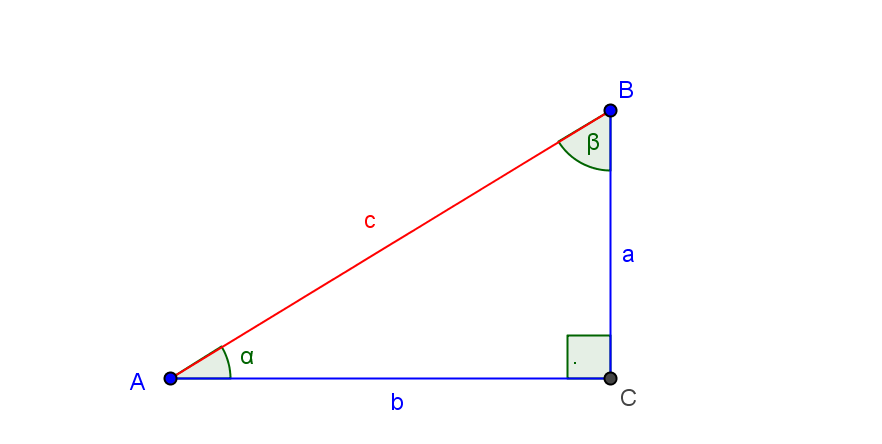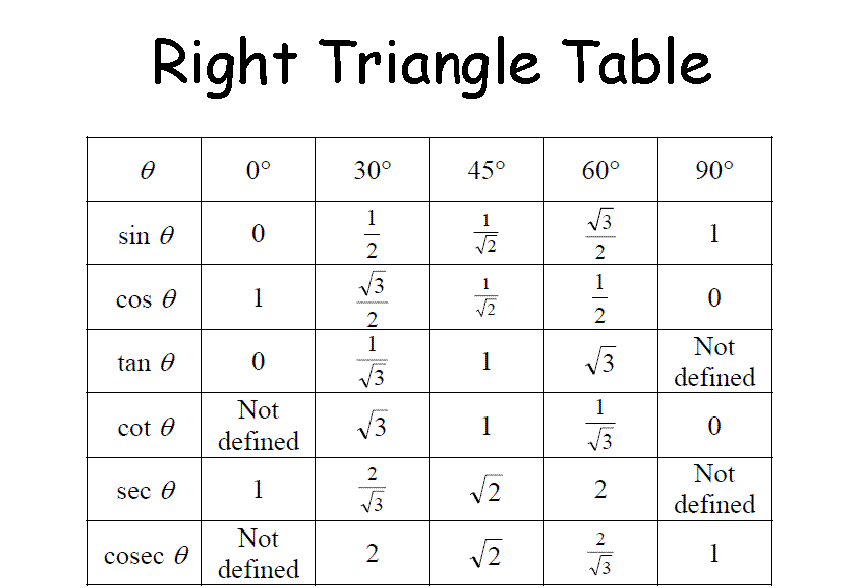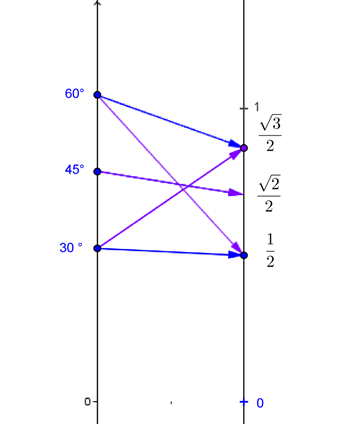(i) Right Triangle Trigonometric Functions
(ii) Unit Circles Trigonometric Functions

(i) Right Triangle Trigonometric Functions
Consider a right triangle $ABC$ with the right angle at vertex $C$, side of length $a$ opposite vertex $A$, with $\angle BAC$  of measure $\alpha$, and side of length $b$ opposite vertex $B$, with $\angle ABC$ of measure $\beta$, and hypotenuse of length $c$.For $\alpha$ measured in degrees, $0^{\circ} < \alpha < 90^{\circ}$ or $\alpha$  measured with radians, $0 < \alpha < \frac {\pi}2$, the following ratios define the value of the trigonometric function for $\alpha$:

 Function Name Abbreviation for Function Name Function Value for $\alpha$ sine sin $\sin(\alpha) = \frac ac$ cosine cos $\cos(\alpha) = \frac bc$ tangent tan $\tan(\alpha) = \frac ab$ cotangent cot $\cot(\alpha) = \frac ba$ secant sec $\sec(\alpha) = \frac cb$ cosecant csc $\csc(\alpha) = \frac ca$

We start with a table for some values for acute angles from the geometry of the 30-60-90 and 45-45-90 triangles and a simple mapping diagram for the sine and cosine functions using degree measure:Here is a dynamic calculator visualizing the triangle trigonometric functions with triangles with $b =1$ and triangles with $c=1$.
 Triangle Trigonometric Functions This is a Java Applet created using GeoGebra from www.geogebra.org - it looks like you don't have Java installed, please go to www.java.com